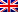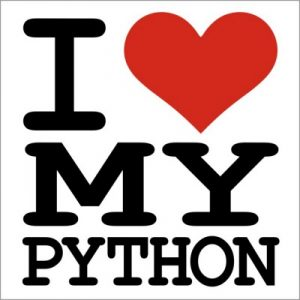#[sCTF 2016] [CODE 130 – I Can’t Get No Satisfaction] Write Up

## Description

I’ve got another task for you involving my little language, Prop!

It consists of the following expressions (denoted `e`):

• Boolean constants, written `true` and `false`.
• Boolean variables, written as any alphabetical string.
• Implies, written `e -> e`.
• Equivalence, written `e <-> e`.
• Negation, written `!e`.
• And, written `e && e`.
• Or, written `e || e`.

You can also parenthesize any expressions or subexpressions (so, you can write `(a || b) && c`). Here’s another example program:

``(a || b) && c && d && (!d || b) || (b -> c) && (d <-> a)``

This time, I’ve determined that the attached program is satisfiable, but I want to know the actual values that make this program satisfiable. Can you find me a satisfying model, please? When you’ve found one, submit a GET request to our server. Any missing keys are considered false.

Here’s an example request:

``http://problems3.2016q1.sctf.io:11420/?a=true&b=true&c=false``

This request would treat `a` and `b` as `true` and `c` and the rest of the variables as `false`. Thus, it’s only necessary to assign the true keys to the value true.

## Resolution

At first glance, we can say that this language looks a lot like python.

Well, okay, maybe that just looks like any language, but what can i say …So our idea was to transform the datas to a true python statement, and then bruteforce the given values to make the statement be True

```f=open('program.prop','rb'
f.close()
```
```Then we transform that text to actual text that a python interpreter might understand. for the most part this is pretty straigtforward :

datas=datas.replace('&&', 'and')
datas=datas.replace('||', 'or')
datas=datas.replace('<->', '==')
datas=datas.replace('true','True')
datas=datas.replace('false','False')
datas=datas.replace('!','not ')```

Implies doesn’t really exist in Python, so this one was a little bit trickier.

A -> B means that if you have A, then you should also have B. so either you don’t have A (A=False) or you have A=True and B=True

this could be simplified by “(not A) or B”

but we now can’t “just” make a simple remplace, as A can be a complexe part, because of all those parenthesis.

so we have to iterate threw all of the datas  :

```#A implies B == (not A) or B
while '-&gt;' in datas:
index=datas.index('-&gt;')
datas=datas[:index]+'or'+datas[index+2:]
#search for the beggening of A
parent_count=0
in_var_name=False
for index2 in range(len(datas[:index][::-1])):
if datas[:index][::-1][index2]=='(':
if parent_count==0 and in_var_name==True :
#found the start
datas=datas[:index-(index2)]+'not '+datas[index-(index2):]
break
parent_count-=1
if parent_count==0 :
#found the start
datas=datas[:index-(index2+1)]+'not '+datas[index-(index2+1):]
break
elif datas[:index][::-1][index2]==')':
if in_var_name==True and parent_count==0 :
#found the start
datas=datas[:index-(index2)]+'not '+datas[index-(index2):]
break
parent_count+=1

elif datas[:index][::-1][index2]==' ':
if in_var_name==True and parent_count==0 :
#found the start
datas=datas[:index-(index2+1)]+'not '+datas[index-(index2+1):]
break
else :
in_var_name=True
```

we have a second problem with the !/not,

``` >> False == not( True)
File "&amp;lt;input&amp;gt;", line 1
False == not( True)
^
SyntaxError: invalid syntax
```

but this is okay :

```>> False == (not( True))
True
```

so we have to re-parenthesis our nots:

```</pre>
<pre>lastindex=0
while datas.find('not',lastindex)>0:
index=datas.find('not',lastindex)
lastindex=index+4
print lastindex
datas=datas[:index]+'('+datas[index:]
#search for the begening of A
parent_count=0
in_var_name=False
for index2 in range(len(datas[index+4:])):
if datas[index+4:][index2]=='(':
parent_count+=1
elif datas[index+4:][index2]==')':
if parent_count==0 :
#found the start
datas=datas[:index+4+(index2)]+') '+datas[index+4+(index2):]
break
parent_count-=1
if parent_count==0 :
#found the start
datas=datas[:index+4+(index2+1)]+') '+datas[index+4+(index2+1):]
break
elif datas[index+4:][index2]==' ':
if in_var_name==True and parent_count==0 :
#found the start
datas=datas[:index+4+(index2+1)]+') '+datas[index+4+(index2+1):]
break
else :
if datas[index+4:][index2:index2+3]!='not' and datas[index+4:][index2-1:index2+3-1]!='not' and datas[index+4:][index2-2:index2+3-2]!='not':
in_var_name=True
#print datas
f=open('raw','wb')
f.write(datas)
f.close()
print 'Done'
return 1
```

We should now have an acceptable Python expression that could be rendered by our interpreter

this can easily be done threw the eval() function that will take a string expression as an input .

Finally, we just have to bruteforce our “Expression” to get correct values so it will be evaluated as True.

this can be done quite quickly with a recursive algorithm :

```<pre>def test(var=[],index=0,value=True):#var containt all our variables (a,b,c,d...z)
var[index]=value
if index==25:#once we have changed all the values, we can evaluate
if the_test(var) ==True:
print "Found one solution!"
for i,v in enumerate(var):
print "%s=%s&"%(alphabet2[i],v),
return True
else : return False

else : #As long as we are not at the 'z' variable, we keep going
if test(var,index+1,True)==True: #next variable in the list should be either True
return True
else :
if test(var,index+1,False)==True: #Or False
return True
return False</pre>
```

`Good work! The flag is sctf{TOO_sat_FOR_you}.`